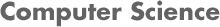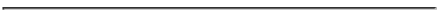### CS 4445 Data Mining and Knowledge Discovery in Databases - A Term 2008  Homework and Project 3: Numeric Predictions

#### PROF. CAROLINA RUIZ

DUE DATES:
• The individual homework assignment is due on Tuesday, Sept. 30 at 1:00 pm, and
• The individual + group project is due on Friday, Oct. 3rd at 12 noon.#### HOMEWORK AND PROJECT OBJECTIVES

The purpose of this project is to construct accurate numeric prediction models for the two datasets under consideration using the following techniques:
• Linear Regression
• Regression Trees
• Model Trees
Also, to gain close understanding of how those methods work, this project also include following those methods by hand on a toy dataset.

• Textbook: Read in great detail the following Sections from your textbook:
• Numeric Predictions: Sections 4.6, 6.5, 5.8.

#### INDIVIDUAL HOMEWORK ASSIGNMENT

See
Solutions by Piotr Mardziel and Amro Khasawneh.

Consider the dataset below. This dataset is an adaptation of the IQ Brain Size Dataset.

```@relation small_twin_dataset

@attribute CCMIDSA numeric 		% Corpus Collasum Surface Area (cm2)
@attribute GENDER {male,female}
@attribute TOTVOL numeric 		% Total Brain Volume (cm3)
@attribute WEIGHT numeric 		% Body Weight (kg)
@attribute FIQ numeric 			% Full-Scale IQ

@data
6.08,	female,	1005,	57.607,		96
5.73,	female,	963,	58.968,		89
7.99,	female,	1281,	63.958,		101
8.42,	female,	1272,	61.69,		103
6.84,	female,	1079,	107.503,	96
6.43,	female,	1070,	83.009,		126
7.6,	male,	1347,	97.524,		94
6.03,	male,	1029,	81.648,		97
7.52,	male,	1204,	79.38,		113
7.67,	male,	1160,	72.576,		124
```
For this homework, we want to predict the FIQ attribute (prediction target) from the other predicting attributes CCMIDSA, GENDER, TOTVOL, and WEIGHT.

1. (15 points) Linear Regression

• (5 points) Write down the general form of the linear regression equation that would result from using linear regression to solve this numeric prediction problem.

• (5 points) Describe in detail the procedure that would be followed by linear regression to find appropriate parameters for this equation.

• (5 points) Run linear regression in the Weka system over this dataset and provide the precise linear regression equation output by Weka (you'll need it for the testing part below). Set the "attributeSelectionMethod" parameter to No Attribute Selection and the "eliminateColinearAttributes" parameter of linear regression to False so that your linear regression formula includes all the predicting attributes.

2. (45 points) Regression Trees and Model Trees
Follow the procedure described in the textbook to construct a model tree and a regression tree to solve this numeric prediction problem. Remember to:

1. (5 points) Start by translating the nominal attribute GENDER into boolean/numeric attributes. This is done by taking the average of the CLASS values associated with each of the gender values Female, Male. Sort them in decresing order by average. Now, create new boolean attributes, one for each possible split of these nominal values in the order listed. After this translation, all the predicting attributes are numeric.

2. (5 points) Sort the values of each attribute in say increasing order. Define a "split point" of an attribute as the midpoint between two subsequent values of the attribute.

3. (5 points) Consider the set of split points of all attributes. Select as the condition for the root node on your tree, the split point that maximizes the value of the following formula:
```   SDR = sd(CLASS over all instances)
- ((k1/n)*sd(CLASS of instances with attribute value below split point)
+ (k2/n)*sd(CLASS of instances with attribute value above split point))

where sd stands for standard deviation.
k1 is the number of instances with attribute value below split point.
k2 is the number of instances with attribute value above split point.
n is the number of instances.
```
To reduce the number of calculations that you need to perform, your HW solutions can be limited to the following split points:
```  binary attributes	CCMIDSA		TOTVOL		WEIGHT
0.5			(6.08+6.43)/2 	(963+1005)/2	(58.968+61.69)/2
(6.84+7.52)/2	(1079+1160)/2	(72.576+79.38)/2
(7.67+7.99)/2	(1160+1204)/2	(83.009+97.524)/2
```
If during the construction of the tree you encounter an attribute such that none of the split points listed above apply to the instances in the node, then use instead all the attribute's split points that apply to that collection of instances.

4. (20 points) Continue the construction of the tree following the same procedure recursively. Remember that for each internal node, the procedure above is applied only to the data instances that belong that that node. You can stop splitting a node when the node contains less than 4 data instances and/or when the standard deviation of the CLASS value of the node's instances is less than 0.05*sda, where sda is the standard deviation of the CLASS attribute over the entire input dataset. See Figure 6.15 on p. 248 of your textbook.

5. (10 points) For each leaf node in the tree:
• Compute the value that would be predicted by that leaf in the case of a Regression Tree.
• Compute the linear regression formula that would be used by that leaf to predict the CLASS value in the case of a Model Tree. In order to find the coefficients of the linear regression formula, run the linear regression method implemented in the Weka system for the appropriate data instances (those that belong to the leaf).

3. (15 points) Testing
Use each of the three numeric predicting models constructed (linear regression equation, model tree and regression tree), to predict the CLASS values (i.e., the FIQ) for each of the test instances below. That is, complete the following table:
```                                                LINEAR        MODEL TREE    REGRESSION TREE
REGRESSION    PREDICTION    PREDICTION
PREDICTION
CCMIDSA	GENDER 	TOTVOL 	WEIGHT 		FIQ

6.22,	female,	1035,	64.184,		87	__________    ___________   __________

6.48,	female,	1034,	62.143,		127	__________    ___________   __________

7.99,	male,	1173,	61.236,		101	__________    ___________   __________

6.59,	male,	1100,	88.452,		114	__________    ___________   __________

LINEAR        MODEL TREE    REGRESSION TREE
REGRESSION    ERROR         ERROR
ERROR

root mean-square error (see p. 178)             __________    ___________   __________

mean absolute error (see p. 178)                __________    ___________   __________

```
SHOW IN DETAIL ALL THE STEPS OF THE PROCESS.

INDIVIDUAL + GROUP PROJECT ASSIGNMENT
[600 points: 100 points per data mining technique per dataset per individual/group parts. See
Project Guidelines for the detailed distribution of these points]

• Project Instructions: THOROUGHLY READ AND FOLLOW THE PROJECT GUIDELINES. These guidelines contain detailed information about how to structure your project, and how to prepare your written and oral reports.

• Individual part and group part of this project:

• Individual Part:
[100 points per technique per dataset] Using the dataset for the individual part (see below), follow the Experiments' Guidelines described in the Project Guidelines and record your observations and results as described there in the individual section of your written report.

• Group Part:
[100 points per technique per dataset] Using the dataset for the group part (see below), and in collaboration with your group partner, follow the Experiments' Guidelines (items 3-8) described in the Project Guidelines and record your joint observations and results as described there in the group section of your written report.

• Data Mining Technique(s): We will run experiment using regression techniques. You need to use:
• Linear Regression (under "functions" in Weka)
• Regression Trees: M5P (under "trees" in Weka) with BuildRegressionTree=True
• Model Trees: M5P (under "trees" in Weka) with BuildRegressionTree=False
on each dataset.

• Datasets: In this project, we will use two datasets, one for the individual part and one for the group part of the project:

• Dataset for the Individual Part of the Project:
The Automobile Dataset available from the The University of California Irvine (UCI) Data Repository.
Use the attribute price as the prediction target. After you run experiments predicting this attribute you may, if you wish, run additional experiments using other numeric predicting targets of your choice.

• Dataset for the Group Part of the Project:
A dataset that you choose depending on your and your group partner's own insterests. It should contain enough instances (at least 200 instances) and several attributes (at least 10). Ideally it should contain a good mix of numeric and nominal attributes. I include below some links to Data Repositories containing multiple datasets to choose from: You can use other data repositories if you wish.

• Performance Metric(s): Use the metrics listed in Table 5.8 (page 178) of the textbook to measure the goodness of your models.
A major part of this project is to try to make sense of these performance metrics and to become familiar with them. When comparing the performance of different models, use tables like Table 5.9 (page 179) of the textbook.

• Miscellaneous: Remember to experiment with and compare the effect of the different parameters of the techniques included in this project:

• Linear Regression: Attribute selection method used; and whether elimination of colinear attributes is used or not.

• M5P: Model vs. Regression trees; prunning vs. non-prunning; smoothing vs. non-smoothing; and variations in the value of minimum number of instances in a leaf.